## Matplotlib Tutorial: Multiple Plots and Double Axes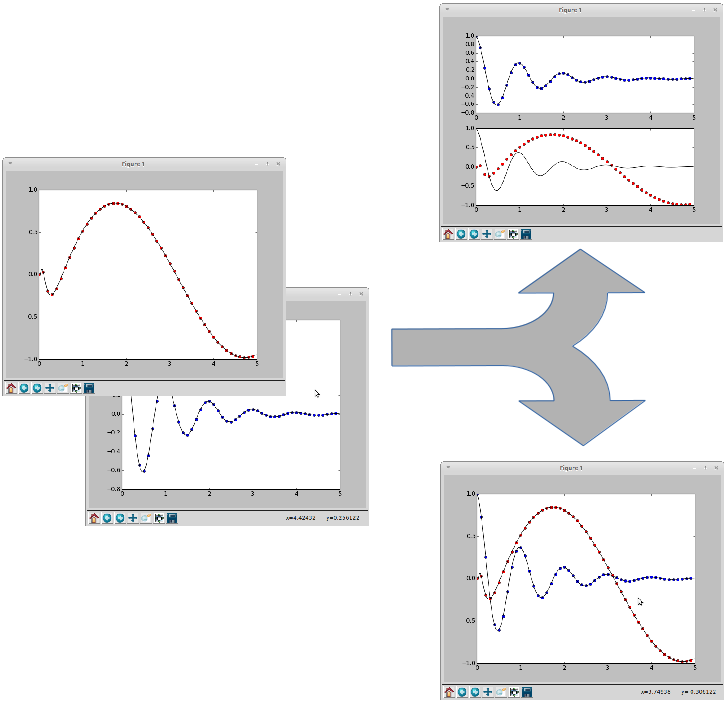We have given so far lots of examples for plotting graphs in the previous chapters of our Python tutorial on Matplotlib. A frequently asked question is how to have multiple plots in one graph?

In the simplest case this might mean, that you have one curve and you want another curve printed over it. This is not a problem, because it will be enough to put the two plots in your scripts, as we have seen before. The more interesting case is, if you want two plots in one window. In one window means that they should be in two separate sublots, i.e. not printed on top of each other. The idea is to have more than one graph in one window and each graph appears in its own subplot.

We will present two different ways to accomplish this goal:

• subplot
• gridspec

We think that the gridspec is the best option, because it is a lot easier to use, if the layout has to be more complex!

### Working with Multiple Figures and Axes

subplot and its parameters:

subplot(nrows, ncols, plot_number)

If a subplot is applied to a figure, the figure will be notionally split into 'nrows' * 'ncols' sub-axes. The parameter 'plot_number' identifies the subplot that the function call has to create. 'plot_number' can range from 1 to a maximum of 'nrows' * 'ncols'.

If the values of the three parameters are less than 10, the function subplot can be called with one int parameter, where the hundreds represent 'nrows', the tens represent 'ncols' and the units represent 'plot_number'. This means: Instead of subplot(2, 3, 4) we can write subplot(234).

In the following example, we "activate" two subplots of a notionally 2x2 grid:

%matplotlib inline

import matplotlib.pyplot as plt

python_course_green = "#476042"
plt.figure(figsize=(6, 4))
plt.subplot(221) # equivalent to: plt.subplot(2, 2, 1)

plt.text(0.5, # x coordinate, 0 leftmost positioned, 1 rightmost
0.5, # y coordinate, 0 topmost positioned, 1 bottommost
'subplot(2,2,1)', # the text which will be printed
horizontalalignment='center', # shortcut 'ha'
verticalalignment='center', # shortcut 'va'
fontsize=20, # can be named 'font' as well
alpha=.5 # float (0.0 transparent through 1.0 opaque)
)
plt.subplot(224, axisbg=python_course_green)
plt.text(0.5, 0.5,
'subplot(2,2,4)',
ha='center', va='center',
fontsize=20,
color="y")

The above code returned the following output:
<matplotlib.text.Text at 0x7f8eae650c50>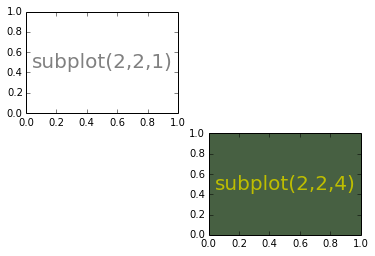For our purposes, we don't need to the ticks on the axes. We can get rid of them by setting them to an emtpy tuple and adding the following code lines to our Python script:

 plt.xticks(()) plt.yticks(()) 

import matplotlib.pyplot as plt

python_course_green = "#476042"
plt.figure(figsize=(6, 4))
plt.subplot(221) # equivalent to: plt.subplot(2, 2, 1)
plt.xticks(())
plt.yticks(())
plt.text(0.5, # x coordinate, 0 leftmost positioned, 1 rightmost
0.5, # y coordinate, 0 topmost positioned, 1 bottommost
'subplot(2,2,1)', # the text which will be printed
horizontalalignment='center', # shortcut 'ha'
verticalalignment='center', # shortcut 'va'
fontsize=20, # can be named 'font' as well
alpha=.5 # float (0.0 transparent through 1.0 opaque)
)
plt.subplot(224, axisbg=python_course_green)
plt.xticks(())
plt.yticks(())
plt.text(0.5, 0.5,
'subplot(2,2,4)',
ha='center', va='center',
fontsize=20,
color="y")

The above code returned the following result:
<matplotlib.text.Text at 0x7f8eae4f3ac8>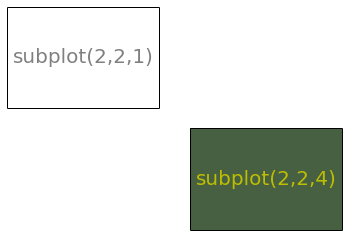Even though the previous approach is acceptable, it is stylistically more appropriate to use an object oriented approach by using instances of the Figure class. We demonstrate this by rewriting the previous example. IN this case, we have to apply the "add_subplot" method to the Figure object.

We recommend reading the chapters on OOP of our Python Tutorial, if you are not familar with object oriented programming:

The revised version of our code looks like this:

import matplotlib.pyplot as plt

python_course_green = "#476042"
fig = plt.figure(figsize=(6, 4))
sub1 = fig.add_subplot(221) # equivalent to: plt.subplot(2, 2, 1)

sub1.text(0.5, # x coordinate, 0 leftmost positioned, 1 rightmost
0.5, # y coordinate, 0 topmost positioned, 1 bottommost
'subplot(2,2,1)', # the text which will be printed
horizontalalignment='center', # shortcut 'ha'
verticalalignment='center', # shortcut 'va'
fontsize=20, # can be named 'font' as well
alpha=.5 # float (0.0 transparent through 1.0 opaque)
)
sub2 = fig.add_subplot(224, axisbg=python_course_green)
sub2.text(0.5, 0.5,
'subplot(2,2,4)',
ha='center', va='center',
fontsize=20,
color="y")

The previous Python code returned the following result:
<matplotlib.text.Text at 0x7f8eae48b320>Let us get rid of the ticks again. This time we cannot use plt.xticks(()) and plt.yticks(()). We have to use the set_xticks(()) and set_yticks(()) methods instead.

import matplotlib.pyplot as plt

python_course_green = "#476042"
fig = plt.figure(figsize=(6, 4))
sub1 = fig.add_subplot(221) # equivalent to: plt.subplot(2, 2, 1)
sub1.set_xticks([])
sub1.set_yticks([])
sub1.text(0.5, # x coordinate, 0 leftmost positioned, 1 rightmost
0.5, # y coordinate, 0 topmost positioned, 1 bottommost
'subplot(2,2,1)', # the text which will be printed
horizontalalignment='center', # shortcut 'ha'
verticalalignment='center', # shortcut 'va'
fontsize=20, # can be named 'font' as well
alpha=.5 # float (0.0 transparent through 1.0 opaque)
)
sub2 = fig.add_subplot(224, axisbg=python_course_green)
sub2.set_xticks([])
sub1.set_yticks([])
sub2.text(0.5, 0.5,
'subplot(2,2,4)',
ha='center', va='center',
fontsize=20,
color="y")

The above code returned the following output:
<matplotlib.text.Text at 0x7f8eae3c3ba8>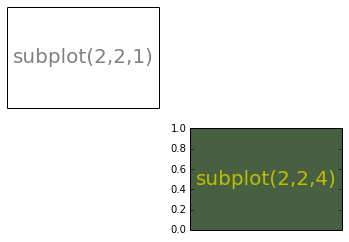Activating all subplot of the 2x2 grid looks like this:

import matplotlib.pyplot as plt

python_course_green = "#476042"
fig = plt.figure(figsize=(6, 4))
sub1 = plt.subplot(2, 2, 1)
sub1.set_xticks(())
sub1.set_yticks(())
sub1.text(0.5, 0.5, 'subplot(2,2,1)', ha='center', va='center',
size=20, alpha=.5)

sub2 = plt.subplot(2, 2, 2)
sub2.set_xticks(())
sub2.set_yticks(())
sub2.text(0.5, 0.5, 'subplot(2,2,2)', ha='center', va='center',
size=20, alpha=.5)

sub3 = plt.subplot(2, 2, 3)
sub3.set_xticks(())
sub3.set_yticks(())
sub3.text(0.5, 0.5, 'subplot(2,2,3)', ha='center', va='center',
size=20, alpha=.5)

sub4 = plt.subplot(2, 2, 4, axisbg=python_course_green)
sub4.set_xticks(())
sub4.set_yticks(())
sub4.text(0.5, 0.5, 'subplot(2,2,4)', ha='center', va='center',
size=20, alpha=.5, color="y")

fig.tight_layout()
plt.show()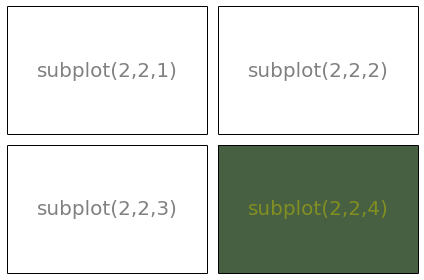The previous examples are solely showing how to create a subplot design. Usually, you want to write Python programs using Matplotlib and its subplot features to depict some graphs. We will demonstrate how to populate the previous subplot design with some example graphs:

import numpy as np
from numpy import e, pi, sin, exp, cos
import matplotlib.pyplot as plt

def f(t):
return exp(-t) * cos(2*pi*t)

def fp(t):
return -2*pi * exp(-t) * sin(2*pi*t) - e**(-t)*cos(2*pi*t)

def g(t):
return sin(t) * cos(1/(t+0.1))

def g(t):
return sin(t) * cos(1/(t))

python_course_green = "#476042"
fig = plt.figure(figsize=(6, 4))

t = np.arange(-5.0, 1.0, 0.1)

sub1 = fig.add_subplot(221) # instead of plt.subplot(2, 2, 1)
sub1.set_title('The function f') # non OOP: plt.title('The function f')
sub1.plot(t, f(t))

sub2 = fig.add_subplot(222, axisbg="lightgrey")
sub2.set_title('fp, the derivation of f')
sub2.plot(t, fp(t))

t = np.arange(-3.0, 2.0, 0.02)
sub3 = fig.add_subplot(223)
sub3.set_title('The function g')
sub3.plot(t, g(t))

t = np.arange(-0.2, 0.2, 0.001)
sub4 = fig.add_subplot(224, axisbg="lightgrey")
sub4.set_title('A closer look at g')
sub4.set_xticks([-0.2, -0.1, 0, 0.1, 0.2])
sub4.set_yticks([-0.15, -0.1, 0, 0.1, 0.15])
sub4.plot(t, g(t))

plt.plot(t, g(t))

plt.tight_layout()
plt.show()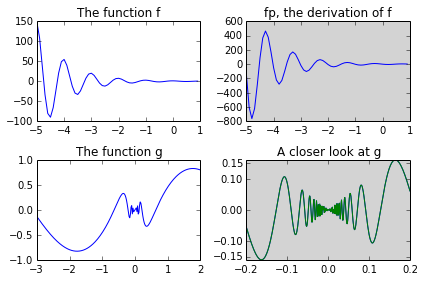Another example:

import  matplotlib.pyplot as plt

X = [ (2,1,1), (2,3,4), (2,3,5), (2,3,6) ]
for nrows, ncols, plot_number in X:
plt.subplot(nrows, ncols, plot_number)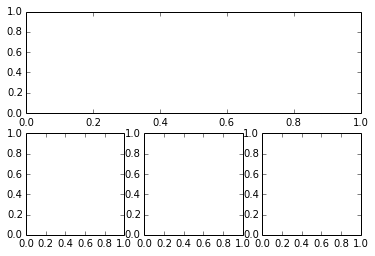The following example shows nothing special. We will remove the xticks and play around with the size of the figure and the subplots. To do this we introduce the keyword paramter figsize of 'figure' and the function 'subplot_adjust' along with its keyword parameters bottom, left, top, right:

import  matplotlib.pyplot as plt

fig =plt.figure(figsize=(6,4))
fig.subplots_adjust(bottom=0.025, left=0.025, top = 0.975, right=0.975)

X = [ (2,1,1), (2,3,4), (2,3,5), (2,3,6) ]
for nrows, ncols, plot_number in X:
sub = fig.add_subplot(nrows, ncols, plot_number)
sub.set_xticks([])
sub.set_yticks([])Alternative Solution:

As the first three three elements of 2x3 grid have to be joined, we can choose a tuple notation, inour case (1,3) in (2,3,(1,3)) to define that the first three elements of a notional 2x3 grid are joined:

import  matplotlib.pyplot as plt

fig =plt.figure(figsize=(6,4))
fig.subplots_adjust(bottom=0.025, left=0.025, top = 0.975, right=0.975)

X = [ (2,3,(1,3)), (2,3,4), (2,3,5), (2,3,6) ]
for nrows, ncols, plot_number in X:
sub = fig.add_subplot(nrows, ncols, plot_number)
sub.set_xticks([])
sub.set_yticks([])## Exercise

How can you create a subplotdesign of a 3x2 design, where the complete first column is spanned?

Solution:

import  matplotlib.pyplot as plt

X = [ (1,2,1), (3,2,2), (3,2,4), (3,2,6) ]
for nrows, ncols, plot_number in X:
plt.subplot(nrows, ncols, plot_number)
plt.xticks([])
plt.yticks([])## Exercise

Create a subplot layout for the following design:Solution:

import  matplotlib.pyplot as plt

X = [  (4,2,1),(4,2,2), (4,2,3), (4,2,5), (4,2,(4,6)), (4,1,4)]
plt.subplots_adjust(bottom=0, left=0, top = 0.975, right=1)
for nrows, ncols, plot_number in X:
plt.subplot(nrows, ncols, plot_number)
plt.xticks([])
plt.yticks([])

plt.show()### Subplots with gridspec

'matplotlib.gridspec' contains a class GridSpec. It can be used as an alternative to subplot to specify the geometry of the subplots to be created. The basic idea behind GridSpec is a 'grid'. A grid is set up with a number of rows and columns. We have to define after this, how much of the grid a subplot should span.

The following example shows the the trivial or simplest case, i.e. a 1x1 grid

import matplotlib.pyplot as plt
from matplotlib.gridspec import GridSpec

fig = plt.figure()
gs = GridSpec(1, 1)
ax = fig.add_subplot(gs[0,0])

plt.show()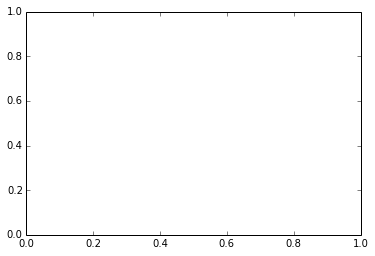We could have used some of the parameters of Gridspec, e.g. we can define, that our graph should begin at 20 % from the bottom and 15 % to the left side of the available figure area:

import matplotlib.pyplot as plt
from matplotlib.gridspec import GridSpec

fig = plt.figure()
gs = GridSpec(1, 1,
bottom=0.2,
left=0.15,
top=0.8)
ax = fig.add_subplot(gs[0,0])

plt.show()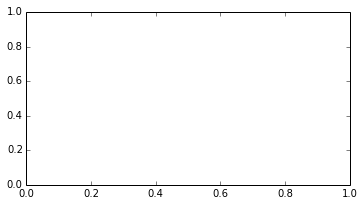The next example shows a complexer example with a more elaborate grid design:

import matplotlib.gridspec as gridspec
import matplotlib.pyplot as pl

pl.figure(figsize=(6, 4))
G = gridspec.GridSpec(3, 3)

axes_1 = pl.subplot(G[0, :])
pl.xticks(())
pl.yticks(())
pl.text(0.5, 0.5, 'Axes 1', ha='center', va='center', size=24, alpha=.5)

axes_2 = pl.subplot(G[1, :-1])
pl.xticks(())
pl.yticks(())
pl.text(0.5, 0.5, 'Axes 2', ha='center', va='center', size=24, alpha=.5)

axes_3 = pl.subplot(G[1:, -1])
pl.xticks(())
pl.yticks(())
pl.text(0.5, 0.5, 'Axes 3', ha='center', va='center', size=24, alpha=.5)

axes_4 = pl.subplot(G[-1, 0])
pl.xticks(())
pl.yticks(())
pl.text(0.5, 0.5, 'Axes 4', ha='center', va='center', size=24, alpha=.5)

axes_5 = pl.subplot(G[-1, -2])
pl.xticks(())
pl.yticks(())
pl.text(0.5, 0.5, 'Axes 5', ha='center', va='center', size=24, alpha=.5)

pl.tight_layout()
pl.show()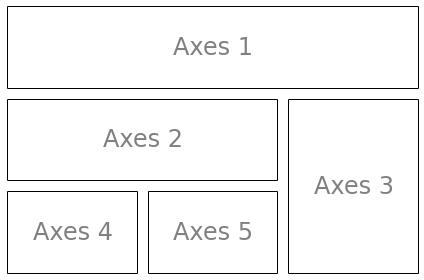We will use now the grid specification from the previous example to populate it with the graphs of some functions:

import matplotlib.gridspec as gridspec
import matplotlib.pyplot as plt
import numpy as np

pl.figure(figsize=(6, 4))
G = gridspec.GridSpec(3, 3)

X = np.linspace(0, 2 * np.pi, 50, endpoint=True)
F1 = 2.8 * np.cos(X)
F2 = 5 * np.sin(X)
F3 = 0.3 * np.sin(X)

axes_1 = plt.subplot(G[0, :])
axes_1.plot(X, F1, 'r-', X, F2)

axes_2 = plt.subplot(G[1, :-1])
axes_2.plot(X, F3)

axes_3 = plt.subplot(G[1:, -1])
axes_3.plot([1,2,3,4], [1,10,100,1000], 'b-')

axes_4 = plt.subplot(G[-1, 0])
axes_4.plot([1,2,3,4], [47, 11, 42, 60], 'r-')

axes_5 = plt.subplot(G[-1, -2])
axes_5.plot([1,2,3,4], [7, 5, 4, 3.8])

plt.tight_layout()
plt.show()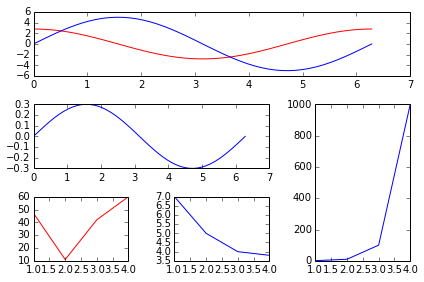### Working with Objects

Matplotlib is programmed and designed -- like Python itself -- in a completely object oriented way. The examples which we have given so far have been very simple, and to keep them as simple as possible, we haven't worked with figure objects for example. Though the objects where automatically created anyway. The advantage of this approach emanate when more than one figure will be used, or when a figure consists of more than one subplot.

In the following example we create a plot in a strictly object-oriented way. We start by creating a new figure instance. We store a reference it in a variable fig. and from it we create a new axis instance axes using the add_axes method in the Figure class instance fig:

import numpy as np
import matplotlib.pyplot as plt

fig = plt.figure()

X = np.arange(0,10)
Y = np.random.randint(1,20, size=10)

left, bottom, width, height = 0.1, 0.1, 0.8, 0.8
axes = fig.add_axes([left, bottom, width, height])

axes.plot(X, Y, 'g')

plt.show(fig)

#axes.set_xlabel('x')
#axes.set_ylabel('y')
#axes.set_title('title');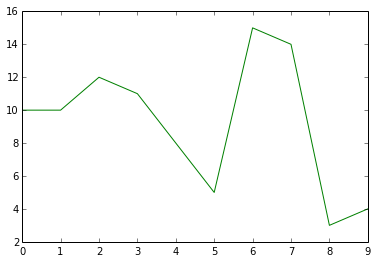Without using explicit instances, the code above looks like this:

import numpy as np
import matplotlib.pyplot as plt

X = np.arange(0,10)
Y = np.random.randint(1,20, size=10)

plt.plot(X, Y, 'g')

plt.show()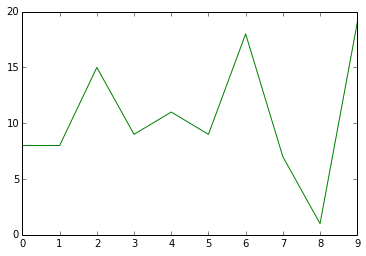### A Plot inside of Another Plot

import numpy as np
import matplotlib.pyplot as plt

fig = plt.figure()

X = [1, 2, 3, 4, 5, 6, 7]
Y = [1, 3, 4, 2, 5, 8, 6]

axes1 = fig.add_axes([0.1, 0.1, 0.9, 0.9]) # main axes
axes2 = fig.add_axes([0.2, 0.6, 0.4, 0.3]) # inset axes

# main figure
axes1.plot(X, Y, 'r')
axes1.set_xlabel('x')
axes1.set_ylabel('y')
axes1.set_title('title')

# insert
axes2.plot(Y, X, 'g')
axes2.set_xlabel('y')
axes2.set_ylabel('x')
axes2.set_title('title inside');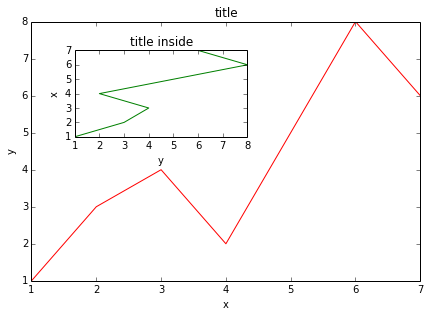### Setting the Plot Range

It's possible to configure the ranges of the axes. This can be done by using the set_ylim and set_xlim methods in the axis object. With axis('tight') we create automatrically "tightly fitted" axes ranges:

import numpy as np
import matplotlib.pyplot as plt

fig, axes = plt.subplots(1, 3, figsize=(10, 4))

x = np.arange(0, 5, 0.25)

axes.plot(x, x**2, x, x**3)
axes.set_title("default axes ranges")

axes.plot(x, x**2, x, x**3)
axes.axis('tight')
axes.set_title("tight axes")

axes.plot(x, x**2, x, x**3)
axes.set_ylim([0, 60])
axes.set_xlim([2, 5])
axes.set_title("custom axes range");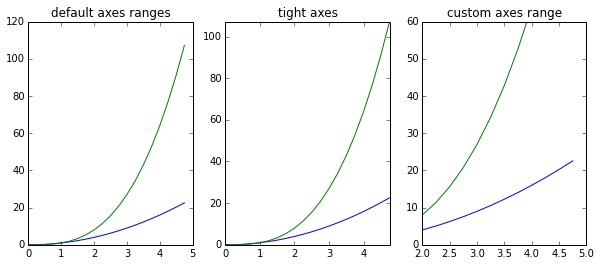### Logarithmic Scale

It is also possible to set a logarithmic scale for one or both axes. This functionality is in fact only one application of a more general transformation system in Matplotlib. Each of the axes' scales are set seperately using set_xscale and set_yscale methods which accept one parameter (with the value "log" in this case):

import numpy as np
import matplotlib.pyplot as plt

fig = plt.figure()
ax = fig.add_subplot(1, 1, 1)

x = np.arange(0, 5, 0.25)

ax.plot(x, x**2, x, x**3)

ax.set_yscale("log")

plt.show()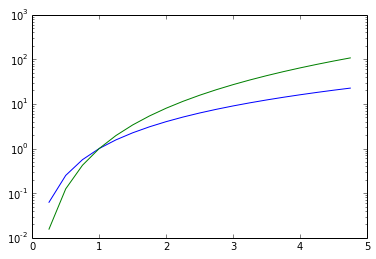import numpy as np
import matplotlib.pyplot as plt

fig, ax1 = plt.subplots()

x = np.arange(1,7,0.1)
ax1.plot(x, 2 * np.pi * x, lw=2, color="blue")
ax1.set_ylabel(r"Circumference $(cm)$", fontsize=16, color="blue")
for label in ax1.get_yticklabels():
label.set_color("blue")

ax2 = ax1.twinx()
ax2.plot(x, np.pi * x ** 2, lw=2, color="darkgreen")
ax2.set_ylabel(r"area $(cm^2)$", fontsize=16, color="darkgreen")
for label in ax2.get_yticklabels():
label.set_color("darkgreen")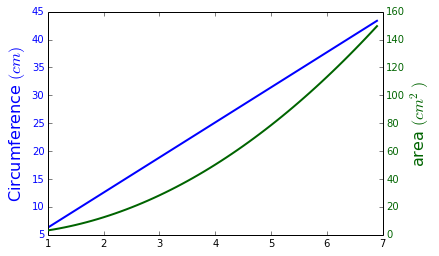The following topics are not directly related to subplotting, but we want to present them to round up the introduction into the basic possibilities of matplotlib. The first one shows how to define grid lines and the second one is quite important. It is about saving plots in image files.

### Grid Lines

import numpy as np
import matplotlib.pyplot as plt

def f(t):
return np.exp(-t) * np.cos(2*np.pi*t)
def g(t):
return np.sin(t) * np.cos(1/(t+0.1))

t1 = np.arange(0.0, 5.0, 0.1)
t2 = np.arange(0.0, 5.0, 0.02)
plt.subplot(212)
plt.plot(t1, g(t1), 'ro', t2, f(t2), 'k')
plt.grid(color='b', alpha=0.5, linestyle='dashed', linewidth=0.5)
plt.show()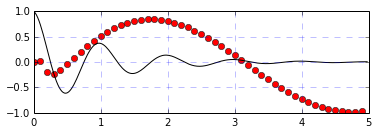### Saving Figures

The savefig method can be used to save figures to a file:

fig.savefig("filename.png")

It is possible to optionally specify the DPI and to choose between different output formats:

fig.savefig("filename.png", dpi=200)

Output can be generated in the formats PNG, JPG, EPS, SVG, PGF and PDF.